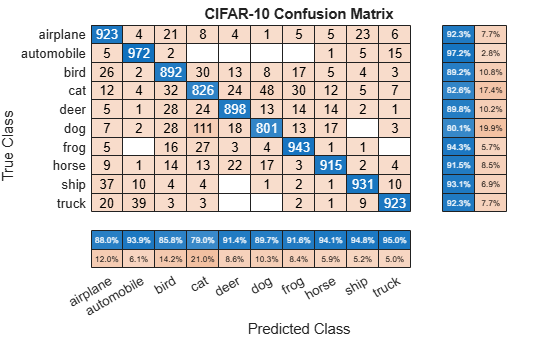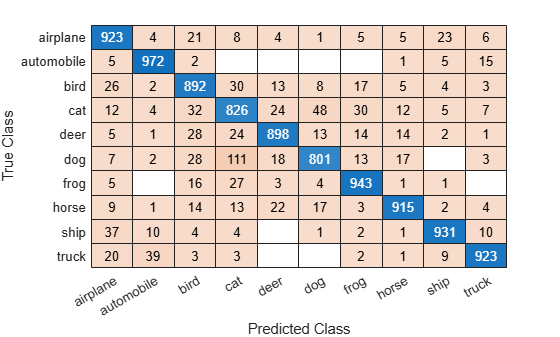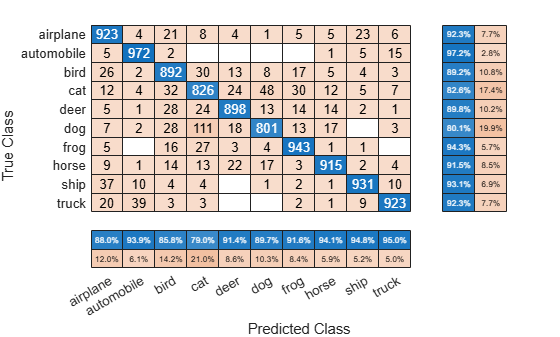Documentation

# confusionchart

Create confusion matrix chart for classification problem

## Syntax

``cm = confusionchart(trueLabels,predictedLabels)``
``cm = confusionchart(m)``
``cm = confusionchart(m,classLabels)``
``cm = confusionchart(parent,___)``
``cm = confusionchart(___,Name,Value)``

## Description

example

````cm = confusionchart(trueLabels,predictedLabels)` creates a confusion matrix chart from true labels `trueLabels` and predicted labels `predictedLabels` and returns a `ConfusionMatrixChart` object. The rows of the confusion matrix correspond to the true class and the columns correspond to the predicted class. Diagonal and off-diagonal cells correspond to correctly and incorrectly classified observations, respectively. Use `cm` to modify the confusion matrix chart after it is created. For a list of properties, see ConfusionMatrixChart Properties.```

example

````cm = confusionchart(m)` creates a confusion matrix chart from the numeric confusion matrix `m`. Use this syntax if you already have a numeric confusion matrix in the workspace.```

example

````cm = confusionchart(m,classLabels)` specifies class labels that appear along the x-axis and y-axis. Use this syntax if you already have a numeric confusion matrix and class labels in the workspace.```
````cm = confusionchart(parent,___)` creates the confusion chart in the figure, panel, or tab specified by `parent`.```
````cm = confusionchart(___,Name,Value)` specifies additional `ConfusionMatrixChart` properties using one or more name-value pair arguments. Specify the properties after all other input arguments. For a list of properties, see ConfusionMatrixChart Properties.```

## Examples

collapse all

Load a sample of predicted and true labels for a classification problem. `trueLabels` is the true labels for an image classification problem and `predictedLabels` is the predictions of a convolutional neural network.

`load('Cifar10Labels.mat','trueLabels','predictedLabels');`

Create a confusion matrix chart.

```figure cm = confusionchart(trueLabels,predictedLabels);```Modify the appearance and behavior of the confusion matrix chart by changing property values. Add column and row summaries and a title. A column-normalized column summary displays the number of correctly and incorrectly classified observations for each predicted class as percentages of the number of observations of the corresponding predicted class. A row-normalized row summary displays the number of correctly and incorrectly classified observations for each true class as percentages of the number of observations of the corresponding true class.

```cm.ColumnSummary = 'column-normalized'; cm.RowSummary = 'row-normalized'; cm.Title = 'CIFAR-10 Confusion Matrix';```You can use `confusionchart` to create a confusion matrix chart from a numeric confusion matrix.

Load a sample confusion matrix `m` and the associated class labels `classLabels`.

```load('Cifar10ConfusionMat.mat','m','classLabels'); m```
```m = 10×10 923 4 21 8 4 1 5 5 23 6 5 972 2 0 0 0 0 1 5 15 26 2 892 30 13 8 17 5 4 3 12 4 32 826 24 48 30 12 5 7 5 1 28 24 898 13 14 14 2 1 7 2 28 111 18 801 13 17 0 3 5 0 16 27 3 4 943 1 1 0 9 1 14 13 22 17 3 915 2 4 37 10 4 4 0 1 2 1 931 10 20 39 3 3 0 0 2 1 9 923 ```
`classLabels`
```classLabels = 10x1 categorical array airplane automobile bird cat deer dog frog horse ship truck ```

Create a confusion matrix chart from the numeric confusion matrix and the class labels.

`cm = confusionchart(m,classLabels);`Load a sample of predicted and true labels for a classification problem. `trueLabels` are the true labels for an image classification problem and `predictedLabels` are the predictions of a convolutional neural network. Create a confusion matrix chart with column and row summaries

```load('Cifar10Labels.mat','trueLabels','predictedLabels'); figure cm = confusionchart(trueLabels,predictedLabels, ... 'ColumnSummary','column-normalized', ... 'RowSummary','row-normalized');```To sort the classes of the confusion matrix by class-wise recall (true positive rate), normalize the cell values across each row, that is, by the number of observations that have the same true class. Sort the classes by the corresponding diagonal cell values and reset the normalization of the cell values. The classes are now sorted such that the percentages in the blue cells in the row summaries to the right are decreasing.

```cm.Normalization = 'row-normalized'; sortClasses(cm,'descending-diagonal'); cm.Normalization = 'absolute';```To sort the classes by class-wise precision (positive predictive value), normalize the cell values across each column, that is, by the number of observations that have the same predicted class. Sort the classes by the corresponding diagonal cell values and reset the normalization of the cell values. The classes are now sorted such that the percentages in the blue cells in the column summaries at the bottom are decreasing.

```cm.Normalization = 'column-normalized'; sortClasses(cm,'descending-diagonal'); cm.Normalization = 'absolute';```## Input Arguments

collapse all

True labels of classification problem, specified as a categorical vector, numeric vector, string vector, character array, cell array of character vectors, or logical vector. If `trueLabels` is a vector, then each element corresponds to one observation. If `trueLabels` is a character array, then it must be two-dimensional with each row corresponding to the label of one observation.

Predicted labels of classification problem, specified as a categorical vector, numeric vector, string vector, character array, cell array of character vectors, or logical vector. If `predictedLabels` is a vector, then each element corresponds to one observation. If `predictedLabels` is a character array, then it must be two-dimensional with each row corresponding to the label of one observation.

Confusion matrix, specified as a matrix. `m` must be square and its elements must be positive integers. The element `m(i,j)` is the number of times an observation of the `i`th true class was predicted to be of the `j`th class. Each colored cell of the confusion matrix chart corresponds to one element of the confusion matrix `m`.

Class labels of the confusion matrix chart, specified as a categorical vector, numeric vector, string vector, character array, cell array of character vectors, or logical vector. If `classLabels` is a vector, then it must have the same number of elements as the confusion matrix has rows and columns. If `classLabels` is a character array, then it must be two-dimensional with each row corresponding to the label of one class.

Parent container in which to plot, specified as a `Figure`, `Panel`, or `Tab` object.

### Name-Value Pair Arguments

Specify optional comma-separated pairs of `Name,Value` arguments. `Name` is the argument name and `Value` is the corresponding value. `Name` must appear inside quotes. You can specify several name and value pair arguments in any order as `Name1,Value1,...,NameN,ValueN`.

Example: ```cm = confusionchart(trueLabels,predictedLabels,'Title','My Title Text','ColumnSummary','column-normalized')```

### Note

The properties listed here are only a subset. For a complete list, see ConfusionMatrixChart Properties.

Title of the confusion matrix chart, specified as a character vector or string scalar.

Example: ```cm = confusionchart(__,'Title','My Title Text')```

Example: `cm.Title = 'My Title Text'`

Column summary of the confusion matrix chart, specified as one of the following:

OptionDescription
`'off'`Do not display a column summary.
`'absolute'`Display the total number of correctly and incorrectly classified observations for each predicted class.
`'column-normalized'`Display the number of correctly and incorrectly classified observations for each predicted class as percentages of the number of observations of the corresponding predicted class. The percentages of correctly classified observations can be thought of as class-wise precisions (or positive predictive values).
`'total-normalized'`Display the number of correctly and incorrectly classified observations for each predicted class as percentages of the total number of observations.

Example: `cm = confusionchart(__,'ColumnSummary','column-normalized')`

Example: `cm.ColumnSummary = 'column-normalized'`

Row summary of the confusion matrix chart, specified as one of the following:

OptionDescription
`'off'`Do not display a row summary.
`'absolute'`Display the total number of correctly and incorrectly classified observations for each true class.
`'row-normalized'`Display the number of correctly and incorrectly classified observations for each true class as percentages of the number of observations of the corresponding true class. The percentages of correctly classified observations can be thought of as class-wise recalls (or true positive rates).
`'total-normalized'`Display the number of correctly and incorrectly classified observations for each true class as percentages of the total number of observations.

Example: `cm = confusionchart(__,'RowSummary','row-normalized')`

Example: `cm.RowSummary = 'row-normalized'`

Normalization of cell values, specified as one of the following:

OptionDescription
`'absolute'`Display the total number of observations in each cell.
`'column-normalized'`Normalize each cell value by the number of observations that has the same predicted class.
`'row-normalized'`Normalize each cell value by the number of observations that has the same true class.
`'total-normalized'`Normalize each cell value by the total number of observations.

Modifying the normalization of cell values also affects the colours of the cells.

Example: `cm = confusionchart(__,'Normalization','total-normalized')`

Example: `cm.Normalization = 'total-normalized'`